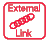# Gravitational Collapse

The source of the energy for star formation is gravitational collapse - this collapse must provide enough energy to heat the gas of the protostar to the ignition point of hydrogen fusion, some 15 million Kelvins. Knowledge of the mass and distribution of the gas cloud permits some fairly detailed modeling, because half of the energy from gravitational collapse goes into kinetic energy according to the virial theorem.

The concept of the Jean's Mass as the critical mass for collapse into a star is an important concept.

Index

Star processes

Galaxy concepts

Reference
Chaisson & McMillan
Ch 19

 HyperPhysics***** Astrophysics R Nave
Go Back

# Virial Theorem

A general theorem from the mathematics of physics becomes a useful part of the picture of gravitational collapse. In the context of gravity it can be applied to a finite collection of particles which interact with each other by gravitational attraction. We can attribute to the collection of particles a total gravitational potential energy and a total kinetic energy. The virial theorem states that

Average kinetic energy = -1/2 x Average potential energy

One application of this theorem would be to a known mass of hydrogen gas in a proto-star. If you had a good estimate of the mass of the gas and could measure a sample of particle velocities to determine the kinetic energy, then you could predict the kinetic energy as the gas cloud underwent gravitational collapse. So for a given radius of collapse, you could make a prediction of the temperature of the hydrogen gas in terms of the kinetic energy and could make a prediction about when it would reach the ignition temperature for hydrogen fusion.

Another potential application is to the question of dark matter. If you examine a system of objects out in space and are able to measure the kinetic energy of the system, then you can infer the gravitational potential energy. If the total mass of all the visible objects is too small to give that amount of gravitational potential energy, then the implication is that there is matter there which you cannot see (dark matter). When this has been done for a variety of types of galaxies, there is strong evidence for dark matter (Baez).

Index

Star processes

Galaxy concepts

ReferenceJohn Baez

 HyperPhysics***** Astrophysics R Nave
Go Back

# Jean's Mass

As a collection of gas begins the process of gravitational collapse, that collapse is opposed by radiation pressure. The "Jean's mass" is the minimum mass that can overcome the radiation pressure for a given energy density in radiation. In the big bang model of the universe, galaxies could not form before the transparency point at which the universe cooled enough for atoms to form, allowing electromagnetic radiation to escape. After that time, the Jean's mass was small enough to allow galaxies and clusters of galaxies to form.

Index

Star processes

Galaxy concepts

References
Rohlf
Ch 19
Harwitt
Sec 12:20

 HyperPhysics***** Astrophysics R Nave
Go Back